## Exercises - Functions and Mapping Diagrams/Transformation Figures

1. Using the formulas provided, find indicated outputs of each function in fully simplified form.

1. $f(x) = 3x+1$     i) $f(5)$,   ii) $f(0)$,   iii) $f(a)$

2. $v(x) = 2^{x-4}$     i) $v(0)$,   ii) $v(4)$,   iii) $v(b)$,   iv) $v(b+4)$

3. $s(x) = \cfrac{|x|}{x}$     i) $s(-2)$,   ii) $s(-5)$,   iii) $s(3)$,   iv) $s(7)$

4. $g(x) = \cfrac{x-2}{x-2}$     i) $g(7)$,   ii) $g(2)$,   iii) $g(a)$

5. $h(x) = \log_2 8x$     i) $h(4)$,   ii) $h(2^a)$

6. $q(x) = x^2 + x$     i) $q(3)$,   ii) $q(b)$,   iii) $q(a+b)$,   iv) $q(x+h)$

7. $j(x) = \sqrt{x} - x \log_2{x}$     i) $j(64)$,   ii) $j(x+1)$,   iii) $j(x^2)$

1. i) $16$,   ii) $1$,   iii) $3a+1$

2. i) $\frac{1}{16}$,   ii) $1$,   iii) $2^{b-4}$,   iv)   $2^b$

3. i) $-1$,   ii) $-1$,   iii) $1$,   iv) $1$

4. i) $1$,   ii) undefined as $2$ is not in the domain of $f$,   iii) $\frac{a-2}{a-2}$ Note that we must not cancel the $(a-2)$ here as doing so would create an expression that is defined when $a=2$, and $g$ is not defined there

5. i) $5$,   ii) $3+a$
Note in (ii) we are safe to simplify all the way to $3+a$ as $8(2^a)$ is always positive for any real value $a$.

6. i) $12$,   ii) $b^2 + b$,   iii) $(a+b)^2 + (a+b)$,   iv) $(x+h)^2 + (x+h)$

7. i) $-376$,   ii) $\sqrt{x+1} - (x+1)\log_2 (x+1)$,   iii) $\sqrt{x^2} - x^2 \log_2 x^2$
Be careful with the last one! You can rewrite $\sqrt{x^2}$ as $|x|$ if desired, but not as $x$. Also, you can't simplify $x^2\log_2 x^2$ to $2x^2\log_2 x$ as if $x$ were negative, the first expression would be defined, but the second would not.

2. Write the following sets in two different ways (i.e., using a special symbol, an explicit list, interval notation, and/or set-bulder notation):

1. All integers that are at least $5$ but less than $8$

2. All real values that are at most $4$, but more than $-2$

3. All real values less than $7$ units away from $3$

4. All negative real values

5. All real values at distance $4$ from $1$

Answers vary, but here are some possibilities:

1. $\{5,6,7\}$, or equivalently $\{x \in \mathbb{Z} \ | \ 5 \le x \lt 8 \}$
Be careful, don't fall into the trap of using interval notation here. Remember the elements of this set are integers, $\mathbb{Z}$ -- real values between these are not included.

2. $(-2,4]$, or equivalently $\{x \in \mathbb{R} \ | \ -2 \lt x \le 4 \}$

3. $(-4,10)$, or equivalently $\{x \in \mathbb{R} \ | \ \textrm{$x$is less than$7$units away from$3$} \}$

4. $(-\infty,0)$, or equivalently $\mathbb{R}_{\lt 0}$

5. $\{-3,5\}$, or equivalently $\{x \in \mathbb{R} \ | \ |x-1| = 4 \}$

3. Find the implicit domain of each function (under the assumption that all outputs of the function must be real values):

1. $g(x) = \cfrac{5x-2}{x+4}$

2. $h(x) = \cfrac{x+1}{\sqrt{x}}$

3. $f(x) = \cfrac{\log x}{x-5}$

4. $t(x) = \log x^2$

5. $r(x) = \sqrt{x} + \sqrt{-x}$

6. $c(x) = \sqrt{x} + \sqrt{-x}$

1. $\{x \in \mathbb{R} \ | \ x \neq -4 \}$     note the denominator is zero when $x=-4$

2. $\{x \in \mathbb{R} \ | \ x > 0 \}$
the square root of a negative is not a real value, and while $\sqrt{0}=0$, we can't divide by zero

3. $\{x \in \mathbb{R} \ | \ x > 0 \textrm{ and } x \neq 5 \}$
seeing $\log x$ requires $x \gt 0$ and $x=5$ creates a division by zero

4. $\{x \in \mathbb{R} \ | \ x \neq 0 \}$
the possible problem with negative $x$ values being under the square root symbol goes away upon squaring them (the result is positive), however we still can't evaluate $\log 0^2 = \log 0$

5. $\{0\}$
if $x \gt 0$ the second square root has an issue; if $x \lt 0$, the first square root has an issue; but $r(0)=0$

6. $\mathbb{R}$
nothing to go wrong here -- we can take the cube root of any real value to get another real value, and we can add any two real values together to get a real value

4. For each, find formulas and domains for   $f+g$,     $f-g$,     $fg$,     $f/g$,     $g/f$,     $f \circ g$,   and   $g \circ f$. Resist the urge to simplify your initial formula -- as doing so improperly can affect the domain..

1. $f(x) = x-5; \quad g(x) = x^2$

2. $f(x) = \sqrt{x}; \quad g(x) = x^2$

3. $f(x) = \cfrac{1}{x-1}; \quad g(x) = \cfrac{1}{x}$

4. $f(x) = \log x; \quad g(x) = 10^x$

5. $f(x) = \log (|x|+1); \quad g(x) = \sqrt{x^4}$

Here are the domains you should see for $f+g$,     $f-g$,     $fg$,     $f/g$,     $g/f$,     $f \circ g$,   and   $g \circ f$, respectively:

1. $\mathbb{R}, \mathbb{R}, \mathbb{R}, \mathbb{R}_{\ne 0}, \mathbb{R}_{\ne 5}, \mathbb{R}, \mathbb{R}$

2. $\mathbb{R}_{\ge 0}, \mathbb{R}_{\ge 0}, \mathbb{R}_{\ge 0}, \mathbb{R}_{\gt 0}, \mathbb{R}_{\gt 0}, \mathbb{R}, \mathbb{R}_{\ge 0}$

3. all are $\{x \in \mathbb{R} \ | \ x \neq 0,1\}$, except the domain of $g \circ f$, which is $\{x \in \mathbb{R} \ | \ x \neq 1\}$

4. the first four are all $\mathbb{R}_{\gt 0}$, while $g/f$,   $f \circ g$, and $g \circ f$ have domains $\{x \in \mathbb{R}_{\gt 0} \ | \ x \neq 1\}$, $\mathbb{R}$, and $\mathbb{R}_{\gt 0}$, respectively

5. all are $\mathbb{R}$, except $f/g$ and $g/f$ which both have domains $\mathbb{R}_{\neq 0}$

5. Identify each function as bijective, injective only, surjective only, or neither injective nor surjective. Argue how you know each conclusion to be true. Then decide if the function has an inverse (in a compositional sense).

1. $f : \mathbb{R} \rightarrow \mathbb{R} : f(x) = x+7$

2. $g : \mathbb{Q} \rightarrow \mathbb{Q} : g(x) = x^2$

3. $h : \mathbb{Q} \rightarrow \mathbb{R} : h(x) = x/5$

4. $p : \mathbb{R} \rightarrow \mathbb{R}_{\ge 0} : h(x) = x^2$

1. bijective, as if $f(a) = f(b)$ then $a+7 = b+7$, which implies $a=b$ (subtract $7$ from both sides). This shows $f$ is injective. Then, note given any output $y \in \mathbb{R}$, we can subtract $7$ from it to produce an $x \in \mathbb{R}$ where $f(x)=y$. Thus, $f$ is surjective. As $f$ is both injective and surjective, it is bijective. Also, being bijective means it has an inverse.

2. neither injective nor surjective. Note that $g(2) = g(-2)$ (where both $2,-2 \in \mathbb{Q}$), so $g$ is not injective. Also, note that $\sqrt{2}$ is not rational, so we won't be able to find an $x \in \mathbb{Q}$ where $g(x) = 2 \in \mathbb{Q}$. Consequently, $g$ is not surjective. Note that either the lack of injectivity or the lack of surjectivity would be enough to conclude $g$ has no inverse.

3. injective only. If $a/5 = b/5$, multiply both sides by $5$ to discover $a=b$, so $h$ is injective. However, if $h(x) = \sqrt{2}$ it must be that $x = 5\sqrt{2}$, which is not rational. Thus, $h$ is not surjective. Because surjectivity fails, $h$ does not have an inverse.

4. surjective only. We can find a real-valued square root of any non-negative real number, so $p$ is surjective. However, there could be more than one. For example, $h(2) = h(-2)$. So $h$ is not injective. Because injectivity fails, $p$ does not have an inverse.

6. Suppose $f$, $g$, and $h$ are functions with domain and co-domain of $\mathbb{R}$. Define new functions $p = f \circ g$ and $q = g \circ h$. If it is also known that $(p \circ h)(3) = 4$, find $(f \circ q)(3)$.

$(f \circ q)(3) = \underbrace{(f \circ (g \circ h))(3) = ((f \circ g) \circ h)(3)}_{\textrm{by associativity}} = (p \circ h)(3) = 4$

7. Determine if the function associated with the given transformation figure is injective. Additionally, make a good guess as to what function is represented, including citing the domain and co-domain in the usual way. In doing this, assume each function is surjective. Assume domains are intervals of real numbers i.e., the spaces between the colored lines shown are just so one can see any other lines overlapping them).

Hints: the function in part (c) has a formula equal to $kx^3$ for some constant $k$, while in part (d) the formula equals $3g(x)$ for some suitable function $g$.

 a)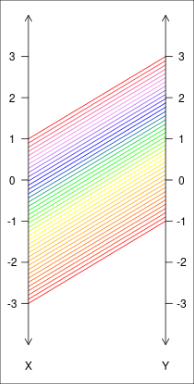b)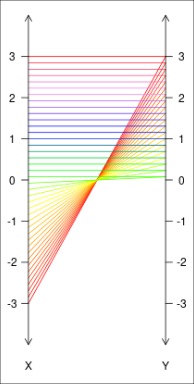c)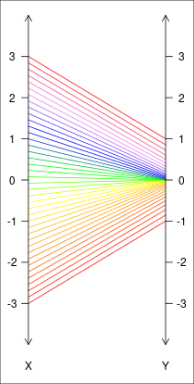d)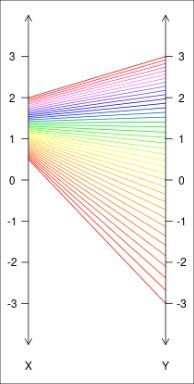1. injective,   $f \ : \ [-3,1] \rightarrow [-1,3], \ \ f(x) = x+2$

2. not injective,   $f \ : \ [-3,3] \rightarrow [0,3], \ \ f(x) = |x|$

3. injective,   $f \ : \ [-3,3] \rightarrow [-1,1], \ \ f(x) = \frac{1}{27} x^3$

4. injective,   $f \ : \ [-\frac{1}{2}, 2] \rightarrow [-3,3], \ \ f(x) = 3\log_2 x$

8. Determine first if each graph represents a function. For those that do, find the domain and range/image, expressing both using interval notation. Also, for any that are functions, indicate if an inverse exists.

Remember that one can express the union of two intervals in interval notation with the "$\cup$" operator. (e.g., $(-\infty,-2] \cup (1,3)$ is the same set as $\{ x \in \mathbb{R} \ | \ x \le -2 \textrm{ or } 1 \lt x \lt 3\}.$ In part (e), assume that the function continues to rise without bound as one moves to the right of $x=8$.

 a)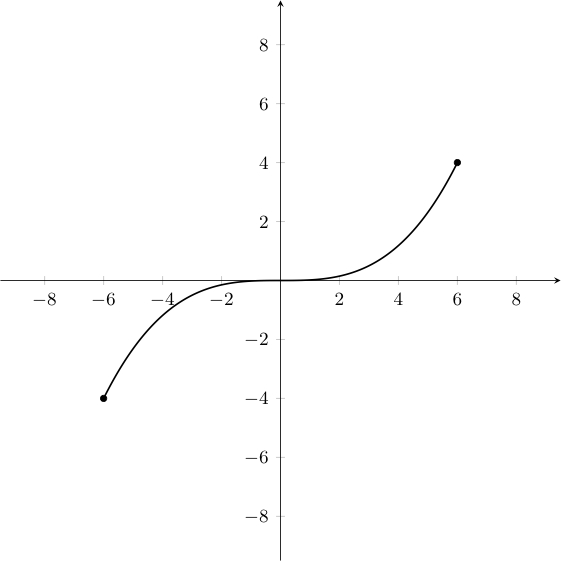b)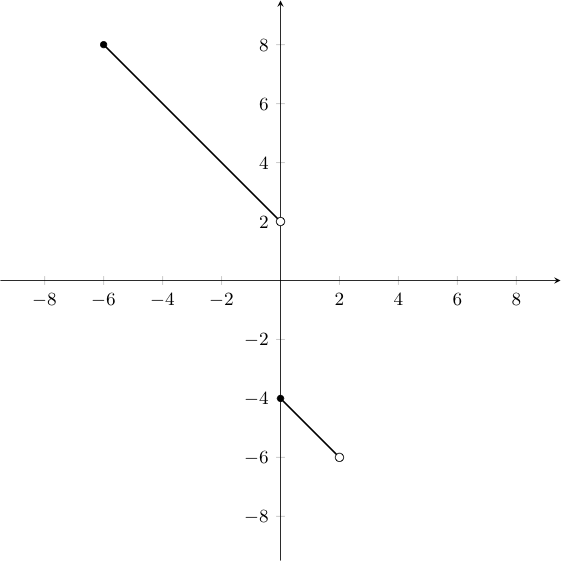c)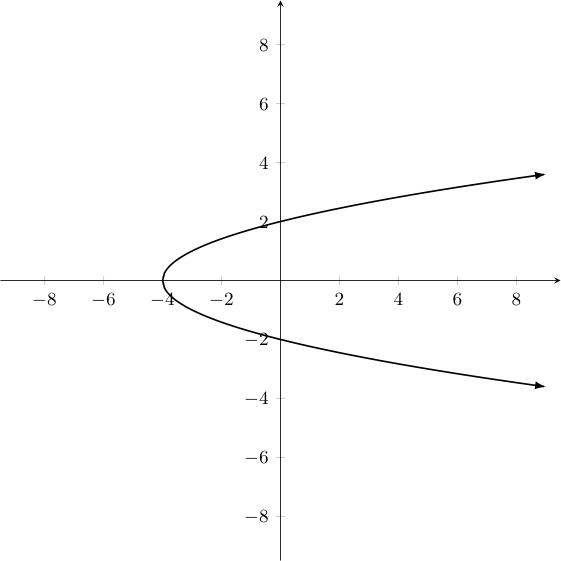d)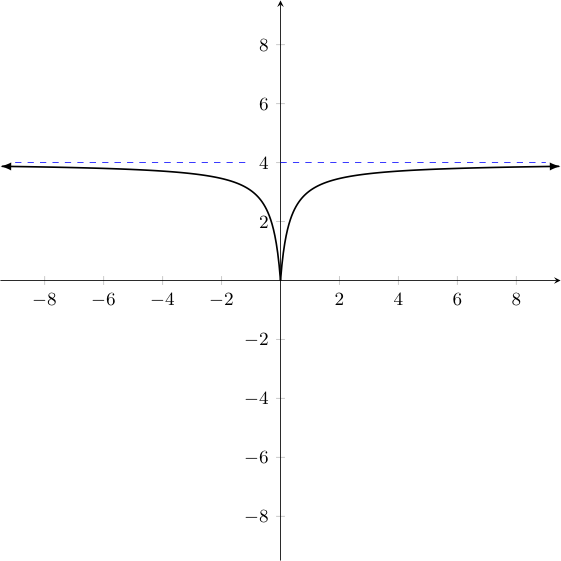e)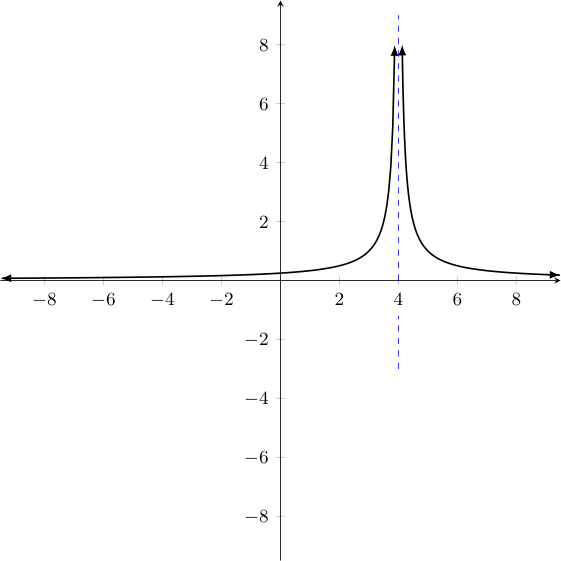f)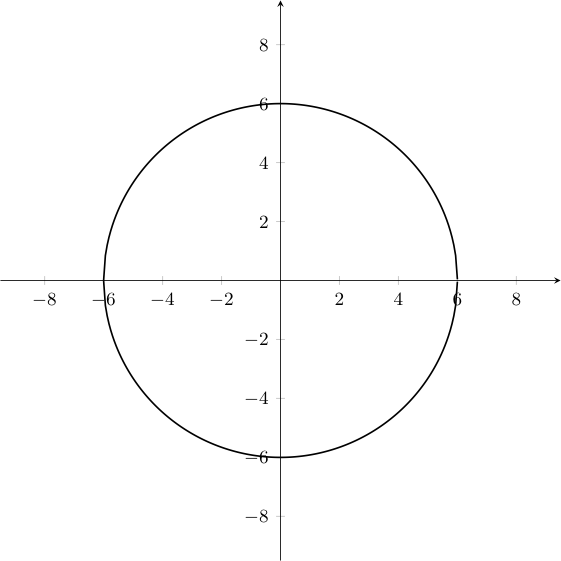g)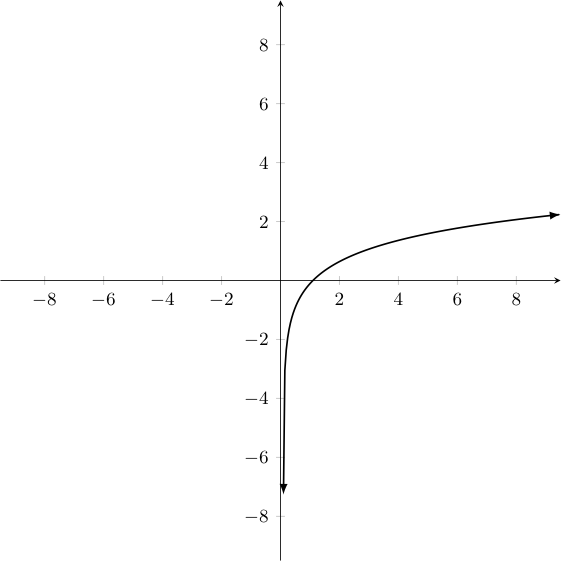h)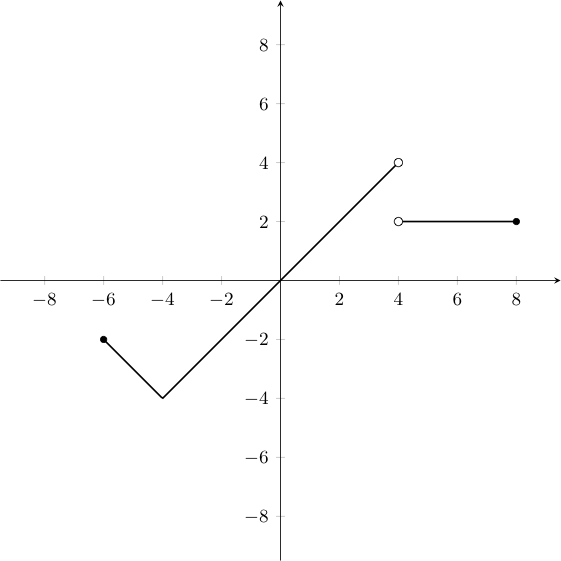1. Domain: $[-6,6]$,   Range/Image: $[-4,4]$; an inverse exists (as it passes the horizontal line test)

2. Domain: $[-6,2)$,   Range/Image: $(-6,-4] \cup (2,8] \cup (4,8]$; an inverse exists (as it passes the horizontal line test)

3. Not a function, it does not pass the vertical line test.

4. Domain: $[-\infty,\infty]$,   Range/Image: $[0,4)$; the graph does not pass the horizontal line test, so the function has no inverse

5. Domain: $(-\infty,4) \cup (4,\infty)$,   Range/Image: $(0,4)$; the graph does not pass the horizontal line test, so the function has no inverse

6. Not a function, it does not pass the vertical line test.

7. Domain: $(0,\infty)$,   Range/Image: $(-\infty,\infty)$; an inverse exists (as it passes the horizontal line test)

8. Domain: $(-6,4) \cup (4,8]$,   Range/Image: $(-4,4]$; the graph does not pass the horizontal line test, so the function has no inverse